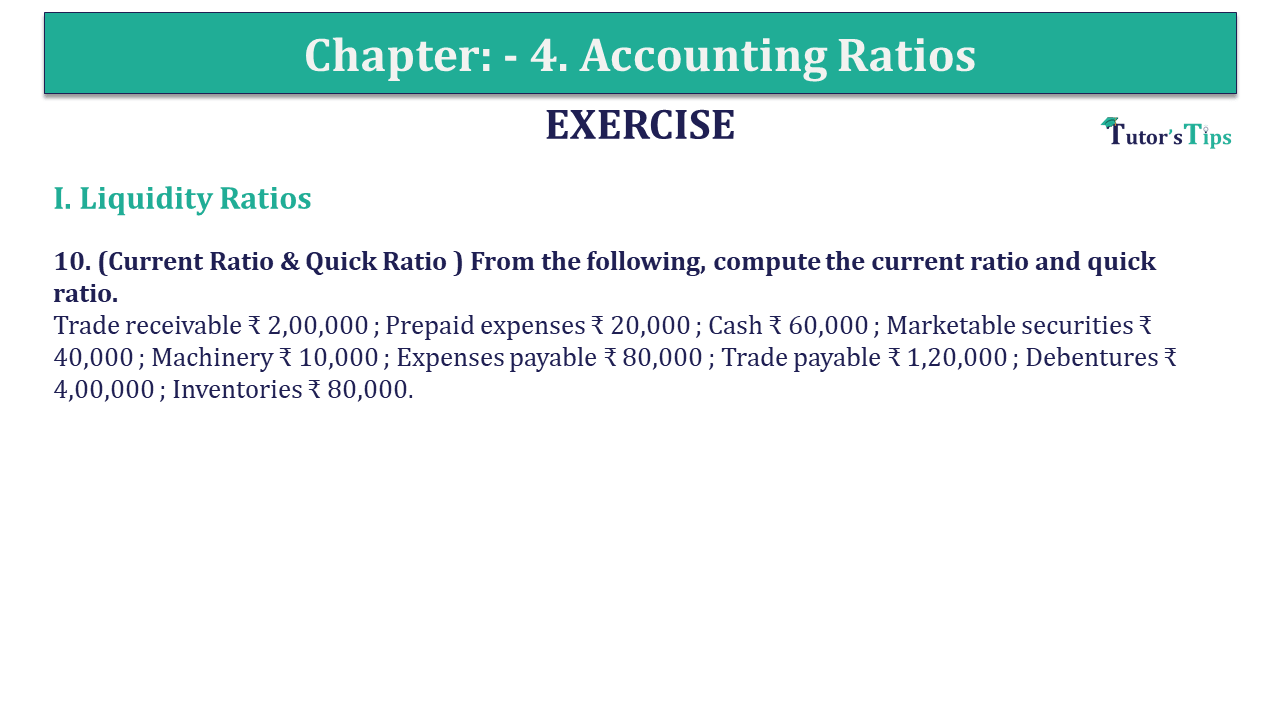# Question 10 Chapter 4 of +2-B – USHA Publication 12 ClassQ-10- CH-4 Book 2 - Usha Pub. +2 Book 2020 - Solution

Question 10 Chapter 4 of +2-B

I. Liquidity Ratios

10. (Current Ratio & Quick Ratio ) From the following, compute the current ratio and quick ratio.
Trade receivable ₹ 2,00,000 ; Prepaid expenses ₹ 20,000 ; Cash ₹ 60,000 ; Marketable securities ₹ 40,000 ; Machinery ₹ 10,000 ; Expenses payable ₹ 80,000 ; Trade payable ₹ 1,20,000 ; Debentures ₹ 4,00,000 ; Inventories ₹ 80,000.

## The solution of Question 10 Chapter 4 of +2-B: –

 Current Assets = Trade receivables + Prepaid expenses + Cash + Marketable Securities + Stock = ₹ 2,00,000 + ₹ 20,000 + ₹ 60,000 + ₹ 40,000 + ₹80,000 Current Assets = ₹ 4,00,000

 Current Liabilities = Trade payable + Expenses payable = ₹ 1,20,000 + ₹ 80,000 Current Liabilities = ₹ 2,00,000

 Current Ratio= Current Assets Current Liabilities

 Current Ratio = ₹ 4,00,000 ₹ 2,00,000 = 2 : 1

 Quick Assets = Current Assets – (Prepaid expenses + Stock) = ₹ 4,00,000 – (₹ 20,000 + ₹80,000) Quick Assets = ₹ 3,00,000 Current Liabilities = ₹ 2,00,000
 Quick Ratio = Quick Assets Current Liabilities = ₹ 3,00,000 ₹ 2,00,000 Quick Ratio = 1.5: 1

What are Liquidity Ratios – Formulas and Examples

Comment if you have any question.

Also, Check out the solved question of previous Chapters: –